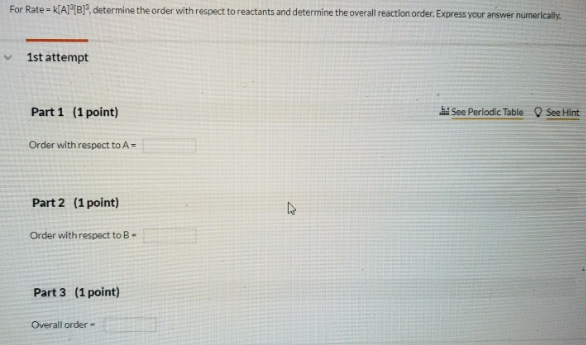# Problem: For Rate = k[A]2[B]3, determine the order with respect to reactants and determine the overall eaction order. Express your answer numerically Part 1 (1 point) Order with respect to A = Part 2 (1 point) Order with respect to B = Part 3 (1 point) Overall order =

###### FREE Expert Solution
84% (378 ratings)###### Problem Details

For Rate = k[A]2[B]3, determine the order with respect to reactants and determine the overall eaction order. Express your answer numerically

Part 1 (1 point)

Order with respect to A =

Part 2 (1 point)

Order with respect to B =

Part 3 (1 point)

Overall order =